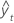Methods and formulas for Single Exponential Smoothing

Select the method or formula of your choice.

Single exponential smoothing

The smoothed (predicted) values are obtained in one of two ways: with an optimal weight generated by Minitab or a weight that you specify.

Optimal ARIMA weight

1. Minitab fits with an ARIMA (0,1,1) model and stores the fits.
2. The smoothed values are the ARIMA model fits, but lagged one time unit.
3. Initial smoothed value (at time one) by backcasting:
• initial smoothed value = [smoothed in period two – α (data in period 1)] / (1 – α)

Notation

TermDescription
1 – α estimates the MA parameter

Specified weight

1. Minitab uses the average of the first six (or N, if N < 6) observations for the initial smoothed value (at time zero). Equivalently, Minitab uses the average of the first six (or N, if N < 6) observations for the initial fitted value (at time one). Fit(i) = Smoothed(i – 1).
2. Subsequent smoothed values are calculated from the formula:
• smoothed value at time t = α (data at t) + (1 – α) (smoothed value at time t – 1)

TermDescription
α weight

Forecasts

The fitted value at time t is the smoothed value at time t – 1. The forecasts are the fitted value at the forecast origin. If you forecast 10 time units ahead, the forecasted value for each time will be the fitted value at the origin. Data up to the origin are used for the smoothing.

In naive forecasting, the forecast for time t is the data value at time t – 1. Perform single exponential smoothing with a weight of one to perform naive forecasting.

Prediction limits

Formula

Based on the mean absolute deviation (MAD). The formulas for the upper and lower limits are:
• Upper limit = Forecast + 1.96 × 1.25 × MAD
• Lower limit = Forecast – 1.96 × 1.25 × MAD

The value of 1.25 is an approximate proportionality constant of the standard deviation to the mean absolute deviation. Hence, 1.25 × MAD is approximately the standard deviation.

MAPE

Mean absolute percentage error (MAPE) measures the accuracy of fitted time series values. MAPE expresses accuracy as a percentage.

Notation

TermDescription
yt actual value at time tfitted value
n number of observations

Mean absolute deviation (MAD) measures the accuracy of fitted time series values. MAD expresses accuracy in the same units as the data, which helps conceptualize the amount of error.

Notation

TermDescription
yt actual value at time tfitted value
n number of observations

MSD

Mean squared deviation (MSD) is always computed using the same denominator, n, regardless of the model. MSD is a more sensitive measure of an unusually large forecast error than MAD.

Notation

TermDescription
yt actual value at time tfitted value
n number of observations
By using this site you agree to the use of cookies for analytics and personalized content.  Read our policy